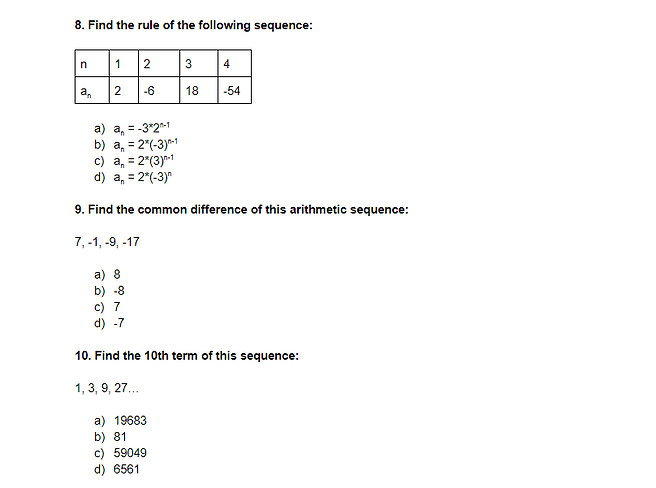Welcome to the guide on Patterns/Sequences

Before you start: This is an introductory lesson, so feel free to skip over concepts you are already comfortable with

Arithmetic/General Sequences:

https://www.mathsisfun.com/algebra/sequences-finding-rule.html (finding rules for sequences)

https://www.chilimath.com/lessons/intermediate-algebra/arithmetic-sequence-formula/ (finding the rule and common difference of arithmetic sequences)

http://www.matematikaria.com/aljabar/sequences-finding-rule.html (more finding rules examples)

https://www.varsitytutors.com/hotmath/hotmath_help/topics/nth-term-of-an-arithmetic-sequence (contains a formula + examples for finding the nth term of an arithmetic sequence)

https://www.youtube.com/watch?v=fifedKPyLLA (a video with step-by-step examples)

https://www.ck12.org/calculus/finding-the-nth-term-given-the-common-difference-and-a-term/lesson/Arithmetic-Sequences-and-Finding-the-nth-Term-Given-the-Common-Difference-and-a-Term-ALG-II/?referrer=concept_details (website with review and examples for finding the nth term of an arithmetic sequence)

Geometric Sequences:

https://www.mathsisfun.com/algebra/sequences-sums-geometric.html (review of geometric sequences)

https://www.ck12.org/book/ck-12-algebra-i-second-edition/section/8.5/ (finding rules for geometric sequences)

https://www.onlinemathlearning.com/geometric-sequences.html (geometric sequences)

https://www.varsitytutors.com/hotmath/hotmath_help/topics/nth-term-of-a-geometric-sequence (finding the nth term of a geometric sequence)

https://www.youtube.com/watch?v=TKtO3C9xpsQ (step-by-step video of how to find the nth term of a geometric sequence)

Mixed Review:

https://www.khanacademy.org/math/algebra/x2f8bb11595b61c86:sequences (This Khan Academy lesson is a great free resource for arithmetic and geometric sequences review, go through the lessons until you are comfortable)

https://brilliant.org/wiki/pattern-recognition-visual-easy-2/ (you will see visual patterns like this on the test, having an understanding of these is helpful)

Practice:

Remember to go back to the learning material if you have any questions

https://www.chilimath.com/lessons/intermediate-algebra/arithmetic-sequence-practice-problems/ (finding rules for an arithmetic sequence)

https://studymaths.co.uk/workout.php?workoutID=20 (the patterns used are somewhat basic, but very helpful if you’re having difficulty finding the nth term)

https://flashmaths.co.uk/viewFlash.php?id=9 (more practice with nth term rules)

https://cdn.kutasoftware.com/Worksheets/Alg2/Arithmetic%20Sequences.pdf (worksheet on arithmetic sequences w/ answer key)

https://www.ixl.com/math/algebra-1/geometric-sequences (practice with geometric sequences)

https://www.mathworksheetsland.com/topics/patterns/1completevisual/practice.pdf (this is a link with some examples of visual patterns)

http://www.visualpatterns.org/ (more examples of visual patterns)

https://www.teach-nology.com/worksheets/math/patterns/numbseq.html (a worksheet on completing a number pattern)

https://brilliant.org/practice/visual-patterns-warmup/ (visual patterns practice)

_____________________________________________________________________________________________________

Check for Understanding (final review):